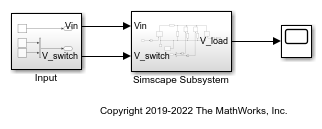# Replacing Piecewise-Constant Resistor

This example shows how to convert a model that is nonlinear due to a Piecewise-Constant Resistor into a switched linear model making it compatible with Simscape to HDL Workflow.

### Introduction

Simscape to HDL Workflow supports conversion of Simscape switched linear models to functionally-equivalent Simulink models that are compatible for HDL code generation. This example uses Piecewise-Constant Resistor which is event based and must be replaced with equivalent switched linear components. Specifically the Piecewise-Constant Resistor contains events that are not supported by the Simscape HDL Workflow Advisor. For more information, see Generate HDL Code for Simscape Models.

Open The Simscape™ Model. In the MATLAB® command prompt, enter:

```nonlinearModel = 'sschdlexVariableResistorExample'; load_system(nonlinearModel) open_system([nonlinearModel,'/Simscape Subsystem']) ```This model is an RLC circuit with a Piecewise-Constant Resistor acting as the resistor or 'load'. For the Piecewise-Constant Resistor, the relationship between voltage V and current I is V=I*R where R is the numerical value presented at the physical signal port R.

To ensure a positive value for the resistance, any value below 1e-6 is replaced by 1e-6. This resistor is Piecewise-Constant because the resistance only changes when the input value differs from the current resistance by more than a set tolerance. Thus, a continuously changing input would be converted to a discrete set of resistances.

In this model the signal going into the Piecewise-Constant Resistor is a step function that changes from 2 to 3 at t=0.1 thus changing the load resistance from 2to 3.To open the Simscape HDL Workflow Advisor at the command-line, enter: sschdladvisor(nonlinearModel)

Run the workflow to the Get state-space parameters task. This task fails because of the presence of the Piecewise-Constant Resistor.### Replace Piecewise-Constant Resistor with switches and constant resistors.

To convert this model to an equivalent switched linear model, replace the Piecewise-Constant Resistor with a set of switches and resistors for each desired value. To open the switched linear version in the MATLAB® command prompt, enter:

```switchedLinearModel = 'sschdlexVariableResistorSwitchedLinearExample'; load_system(switchedLinearModel) open_system(switchedLinearModel) ```The Piecewise-Constant Resistor has been replaced by a resistor and switch for each desired resistance. To recreate the behavior of a load resistance that changes from 2to 3at t=0.1 two resistors are used, one with a resistance of 2and the other with a resistance of 3. By closing and opening the switches the load resistance switches from 2to 3.

### Controlling the Switches

The switches must be turned on and off to provide the correct load resistance. To view the control signals for the switches in the MATLAB® command prompt, enter:

```open_system([switchedLinearModel,'/Input']) ```To achieve the correct resistance, create two step functions. One to open the switch in series with the 2resistor at t=0.1 and another to close the switch in series with the 3resistor at the same time.

Now that the Piecewise-Constant Resistor has been replaced with switched linear components run the Simscape HDL Workflow Advisor and see that all the tasks run to completion.By changing the Piecewise-Constant Resistor to a number of specified resistors that switch on and off the model has been changed to a form that is compatible with the Simscape to HDL workflow.